Next: Other Quantum Algorithms Up: Quantum Algorithms Previous: Overview of Shor's Algorithm   Contents

## Steps to Shor's Algorithm

Shor's algorithm for factoring a given integer n can be broken into some simple steps.

Step 1
Determine if the number n is a prime, a even number, or an integer power of a prime number. If it is we will not use Shor's algorithm. There are efficient classical methods for determining if a integer n belongs to one of the above groups, and providing factors for it if it is. This step would be performed on a classical computer.

Step 2
Pick a integer q that is the power of 2 such that n2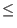q < 2n2. This step would be done on a classical computer.

Step 3
Pick a random integer x that is coprime to n. When two numbers are coprime it means that their greatest common divisor is 1. There are efficient classical methods for picking such an x. This step would be done on a classical computer.

Step 4
Create a quantum register and partition it into two parts, register 1 and register 2. Thus the state of our quantum computer can be given by: |reg1, reg2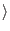. Register 1 must have enough qubits to represent integers as large as q - 1. Register 2 must have enough qubits to represent integers as large as n - 1. The calculations for how many qubits are needed would be done on a classical computer.

Step 5
Load register 1 with an equally weighted superposition of all integers from 0 to q - 1. Load register 2 with all zeros. This operation would be performed by our quantum computer. The total state of the quantum memory register at this point is: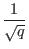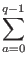| a, 0Step 6
Now apply the transformation xa mod n to for each number stored in register 1 and store the result in register 2. Due to quantum parallelism this will take only one step, as the quantum computer will only calculate x| amod n, where | ais the superposition of states created in step 5. This step is performed on the quantum computer. The state of the quantum memory register at this point is:| a, xa modnStep 7
Measure the second register, and observe some value k. This has the side effect of collapsing register one into a equal superposition of each value a between 0 and q - 1 such that

xa mod n = k

This operation is performed by the quantum computer. The state of the quantum memory register after this step is: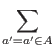| a', kWhere A is the set of a's such that xa mod n = k, and || A|| is the number of elements in that set.

Step 8
Now compute the discrete Fourier transform on register one. The discrete Fourier transform when applied to a state | achanges it in the following manner:

| a=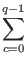| c*e2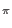iac/q

This step is performed by the quantum computer in one step through quantum parallelism. After the discrete Fourier transform our register is in the state:| c, k*e2ia'c/q

Step 9
Measure the state of register one, call this value m, this integer m has a very high probability of being a multiple of q/r, where r is the desired period. This step is performed by the quantum computer.

Step 10
Take the value m, and on a classical computer do some post processing which calculates r based on knowledge of m and q. In particular:
• m has a high probability of being =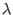*(q/r) whereis an integer
• If we perform floating point division of m/q, and then calculate the best rational approximation to m/q whose denominator is less than or equal to q
• We take this denominator to be a candidate for r.
• If our candidate r is odd we either double it if doing so leads to a value less than q
There are efficient ways to do this post processing on a classical computer.

Step 11
Once you have attained r, a factor of n can be determined by taking gcd(xr/2 + 1, n) and gcd(xr/2 - 1, n). If you have found a factor of n, then stop, if not go to step 4. This final step is done on a classical computer.

Step 11 contains a provision for what to do if Shor's algorithm failed to produce factors of n. There are a few reasons why Shor's algorithm can fail, for example the discrete Fourier transform could be measured to be 0 in step 9, making the post processing in step 10 impossible. The algorithm will sometimes find factors of 1 and n, which is not useful either. For these reasons step 11 must be able to jump back to step 4 to start over. (Williams, Clearwater)Next: Other Quantum Algorithms Up: Quantum Algorithms Previous: Overview of Shor's Algorithm   Contents
Matthew Hayward - Quantum Computing and Shor's Algorithm GitHub Repository